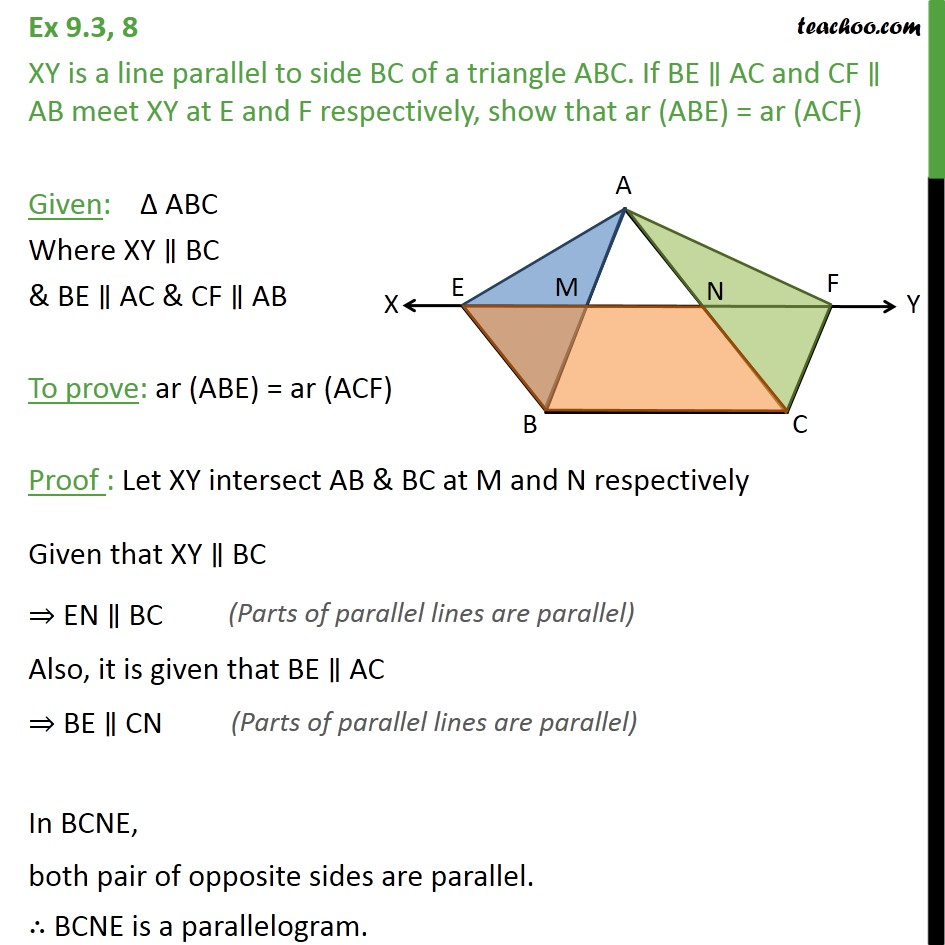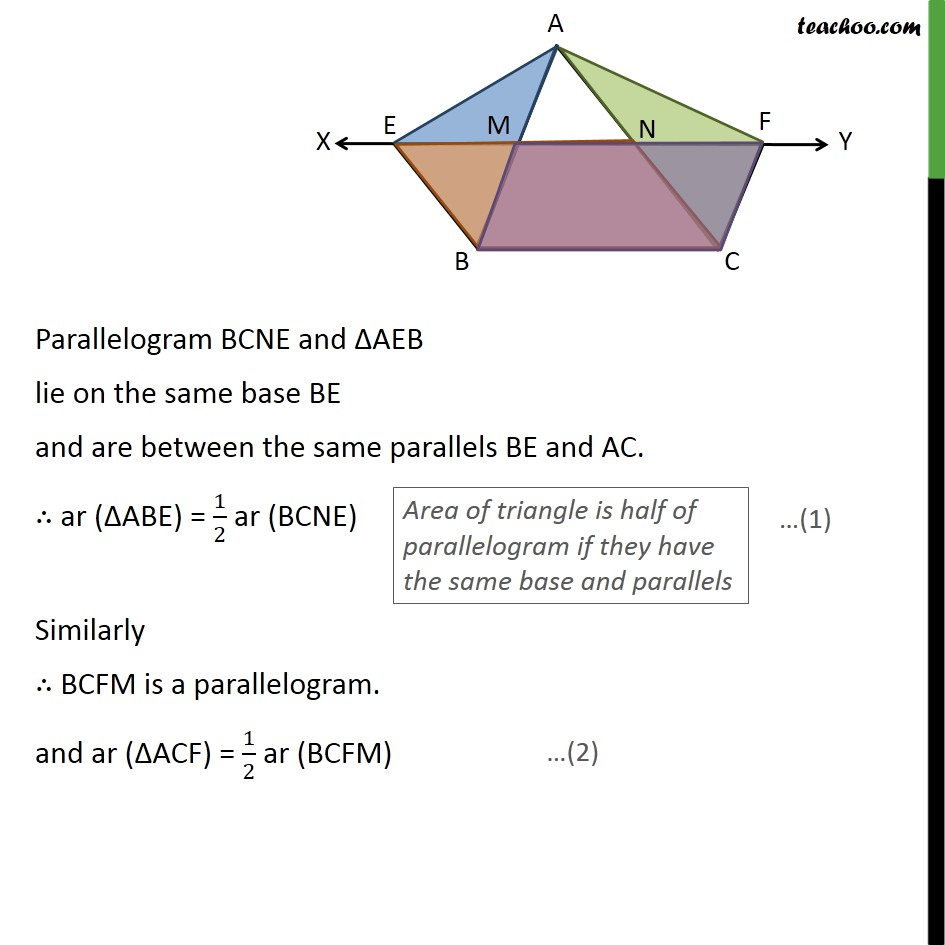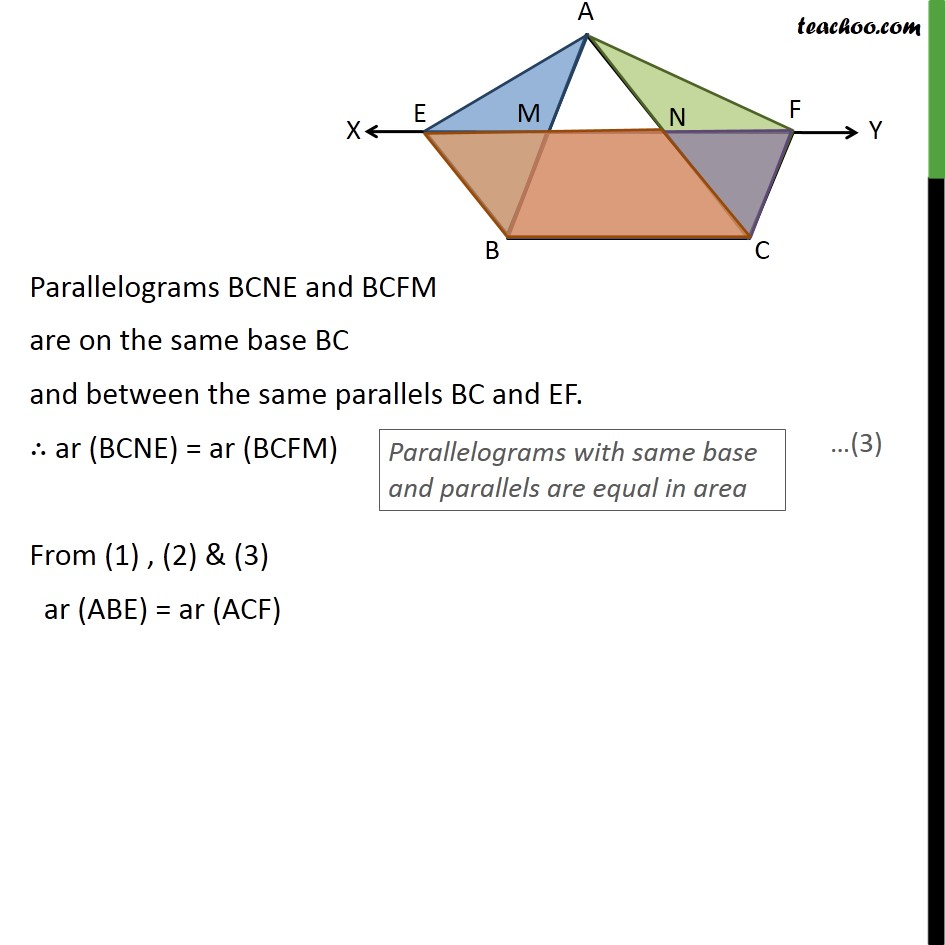Area of Triangles

Areas of Parallelograms and Triangles
Serial order wiseLearn in your speed, with individual attention - Teachoo Maths 1-on-1 Class

### Transcript

Question 8 XY is a line parallel to side BC of a triangle ABC. If BE AC and CF AB meet XY at E and F respectively, show that ar (ABE) = ar (ACF) Given: ABC Where XY BC & BE AC & CF AB To prove: ar (ABE) = ar (ACF) Proof : Let XY intersect AB & BC at M and N respectively Given that XY BC EN BC Also, it is given that BE AC BE CN In BCNE, both pair of opposite sides are parallel. BCNE is a parallelogram. Parallelogram BCNE and AEB lie on the same base BE and are between the same parallels BE and AC. ar ( ABE) = 1/2 ar (BCNE) Similarly BCFM is a parallelogram. and ar ( ACF) = 1/2 ar (BCFM) Parallelograms BCNE and BCFM are on the same base BC and between the same parallels BC and EF. ar (BCNE) = ar (BCFM) From (1) , (2) & (3) ar (ABE) = ar (ACF)